Courses

# Theory Of Machines 2

## 20 Questions MCQ Test Mock Test Series for SSC JE Mechanical Engineering | Theory Of Machines 2

Description
This mock test of Theory Of Machines 2 for Mechanical Engineering helps you for every Mechanical Engineering entrance exam. This contains 20 Multiple Choice Questions for Mechanical Engineering Theory Of Machines 2 (mcq) to study with solutions a complete question bank. The solved questions answers in this Theory Of Machines 2 quiz give you a good mix of easy questions and tough questions. Mechanical Engineering students definitely take this Theory Of Machines 2 exercise for a better result in the exam. You can find other Theory Of Machines 2 extra questions, long questions & short questions for Mechanical Engineering on EduRev as well by searching above.
QUESTION: 1

### The given kinematic link isSolution:

A link is defined as a member or a combination of members of a mechanism, connecting other members and having motion relative to them. Thus, a link may consist of one or more resistant bodies.

Links can be classified into binary, ternary and quaternary depending upon their ends.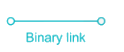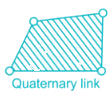QUESTION: 2

### Rack and pinion arrangement is used for:

Solution:

Rack and pinion gears are used to convert rotation into linear motion. The ‘pinion’ is the normal round gear and the ‘rack’ is straight or flat.

A perfect example of this is the steering system on many cars.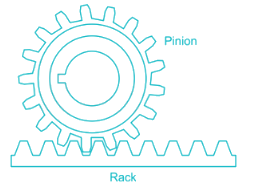QUESTION: 3

### Which of the following mechanisms are examples of force closed kinematic Pairs? A. Cam and roller mechanism B. Door-closing mechanism C. Slider-crank mechanism

Solution:

Self-closed pair:

When the two elements of a pair are connected together mechanically. The contact between the two can be broken only by destruction of at least one of the members.

All lower pairs and some higher pairs are closed pairs. Sliding pairs, turning pairs, spherical pairs and screw pairs are also closed pairs.

Forced-closed pair:

When two links of a pair are in contact either due to force of gravity or some spring action. In this, the links are not held together mechanically e.g. cam and follower pair (because spring is used to keep the motion constrained).

Slider-crank mechanism does not require such force.

QUESTION: 4

In Watt’s Governor, h = ________.

Solution:

For Watt Governor: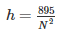i.e height is inversely proportional to (speed)2

QUESTION: 5

The graph of turning moment diagram is drawn between ________.

Solution: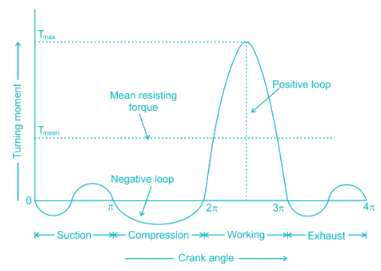The turning moment diagram also known as crank effort diagram.

• It is the graphical representation of the turning moment or torque or crank effort (X-axis) for various position of crank (Y-axis).
• The area under the turning moment diagram gives the work done per cycle.
• The work done per cycle when divided by the crank angle per cycle gives mean torque Tm.
QUESTION: 6

Which of the following term defines the size of the cam?

Solution:

Base Circle: It is the smallest circle, drawn tangential to cam profile. The base circle decides the overall size of the cam and thus is fundamental feature.

Pitch Curve: If we hold the cam fixed and rotate the follower in a direction opposite to that of the cam, then the curve generated by the locus of the trace point is called pitch curve. For a knife edge follower, the pitch curve and the cam profile are same whereas for a roller follower, they are separated by the radius of the roller.

Pitch Point: Pitch point corresponds to the point of maximum pressure angle, and a circle drawn with its centre at the cam centre, to pass through the pitch point, is known as the pitch circle.

Prime Circle: It is the smallest circle that can be drawn from the centre of the cam and tangent to the pitch curve.

QUESTION: 7

For a vibrating system, if the damping factor is unity, then the system is ________ damped.

Solution:

The damping ratio (ζ = c/cc) is a system parameter, that can vary from un-damped (ζ = 0), under-damped (ζ < 1) through critically damped (ζ = 1) to over-damped (ζ > 1)

QUESTION: 8

Gyroscopic effect is not observed in which of the following actions performed by the ships?

Solution:

For the effect of gyroscopic couple to occur, the axis of precession should always be perpendicular to the axis of spin. If, however, the axis of precession becomes parallel to the axis of spin, there will be no effect of the gyroscopic couple acting on the body of the ship.In case of rolling of a ship, the axis of precession (i.e. longitudinal axis) is always parallel to the axis of spin for all positions. Hence, there is no effect of the gyroscopic couple acting on the body of a ship.

QUESTION: 9

What is the condition of complete balancing of reciprocating parts of an engine?

Solution:

Conditions of complete balancing of reciprocating parts of an engine:

• Primary forces must balance i.e., primary force polygon is enclosed.
• Primary couples must balance i.e., primary couple polygon is enclosed.
• Secondary forces must balance i.e., secondary force polygon is enclosed.
• Secondary couples must balance i.e., secondary couple polygon is enclosed.
QUESTION: 10

The Interference or undercutting in involute gears can be avoided by:-

Solution:

Methods of elimination of Gear tooth Interference:

• Use of a larger pressure angle (having a larger pressure angle results in a smaller base circle. As a result, more of the tooth profiles become involute)
• Under-cutting of tooth (A portion of teeth below the base circle is cut off. When teeth are produced by this process, the tip of one tooth of a gear will not contact the non-involute portion of the tooth of other gear)
• Tooth stubbing (In this process a portion of the tip of the teeth is removed, thus preventing that portion of the tip of tooth in contacting the non-involute portion of the other meshing tooth).
• Increasing the number of teeth on the gear can also eliminate the chances of interference.
• Increasing slightly the center distance between the meshing gears would also eliminate interference.
• Tooth profile modification or profile shifting (Using profile shifted gears (gears with non-standard profile) can also be an option to eliminate interference. In profile shifted meshing gears, the addendum on the pinion is shorter compared with standard gears).
QUESTION: 11

If a more stiff spring is used in Hartnell governor, then the governor will be ________.

Solution:

A governor is said to be sensitive when it readily responds to a small change of speed.

Stiffness is inversely proportional to sensitiveness. Therefore, if stiffness is greater, the governor will be less sensitive.

QUESTION: 12

Which one of the following laws is not applicable for a simple pendulum?

Solution:

A simple pendulum is one which can be considered to be a point mass suspended form a string or rod of negligible mass.

The period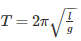So the statement the time period is proportional to its length is wrong.

QUESTION: 13

If C1 is the coefficient of speed fluctuation of a flywheel then the ratio of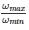will be

Solution:

We know that coefficient of fluctuation of speed (C1) is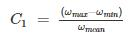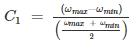Or, Cωmax + Cωmin = 2ωmax – 2ωmin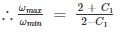QUESTION: 14

A gear set consists of a 16-tooth pinion driving a 40-tooth gear. The module is 12 mm. The addendum and dedendum are 12 mm and 15 mm, respectively and the gears are cut using 20° pressure angle. The centre distance is

Solution: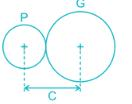TP = 16, TG = 40, m = 12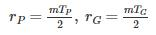C = rP + rG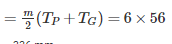336 mm

QUESTION: 15

A crank of radius 15 cm is rotating at 50 rpm with an angular acceleration of 60 rad/sec2. The tangential acceleration of the crank is about

Solution:

Tangential Acceleration at = rα

α = Angular acceleration = 60 rad/s2

r = radius of crank = 15 cm

∴ at = rα = 60 × 0.15 = 9 m/s

QUESTION: 16

The kinetic energy of a flywheel, having moment of inertia I and angular speed ‘ω’, is given by

Solution:

Kinetic energy of the flywheel is given by: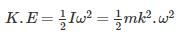I is mass moment of inertia of the flywheel about its axis of rotation in kg-m2 = m.k2

QUESTION: 17

Angle of dwell of cam is defined as the angle

Solution:

Angle of Dwell: It is the angle through which the cam turns while the follower remains stationary at the highest or the lowest position.

QUESTION: 18

The number of instantaneous centres of rotation in a slider-crank quick-return mechanism is

Solution:

Crank and slotted lever quick return motion mechanism is an inversions of Single Slider Crank Chain which is a modification of the basic four bar chain.

Number of instantaneous centres: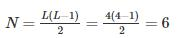where L is number of links.

QUESTION: 19

What will be the locus of a point on a thread unwound from a cylinder?

Solution:

If a straight line is rolled round a circle or a polygon without slipping or sliding, points on line will trace out involutes.

Or Involute of a circle is a curve traced out by a point on a tights string unwound or wound from or on the surface of the cylinder.

QUESTION: 20

Transmissibility is defined as

Solution:

In vibration isolation system, the ratio of the force transmitted to the force applied is known as the isolation factor or transmissibility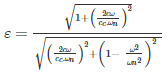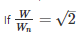then ϵ = 1 for all values of damping factor c/cc.